Скачать презентацию Intro to Price index refer to the

• Количество слайдов: 16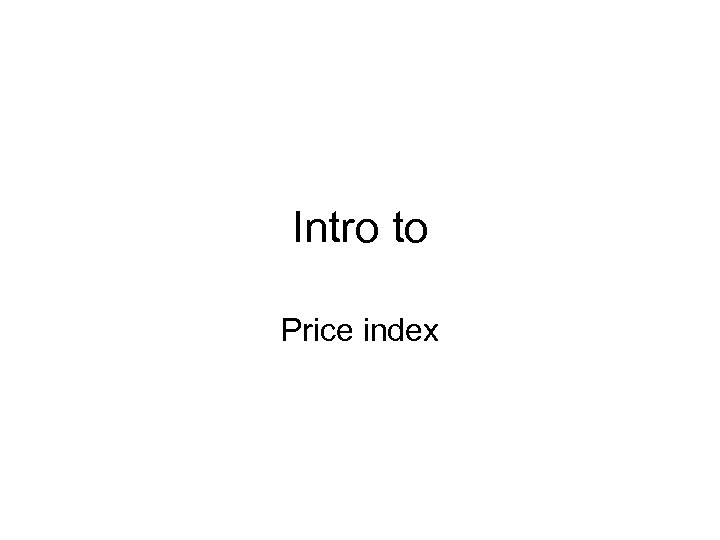Intro to Price index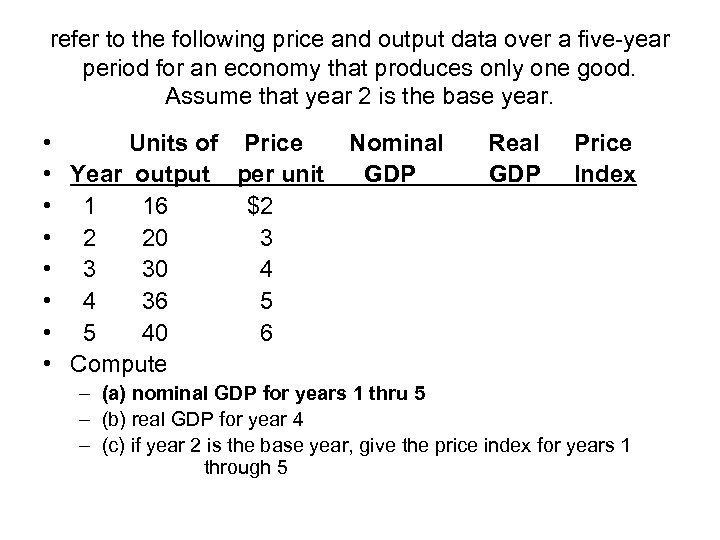refer to the following price and output data over a five-year period for an economy that produces only one good. Assume that year 2 is the base year. • Units of Price • Year output per unit • 1 16 \$2 • 2 20 3 • 3 30 4 • 4 36 5 • 5 40 6 • Compute Nominal GDP Real GDP Price Index – (a) nominal GDP for years 1 thru 5 – (b) real GDP for year 4 – (c) if year 2 is the base year, give the price index for years 1 through 5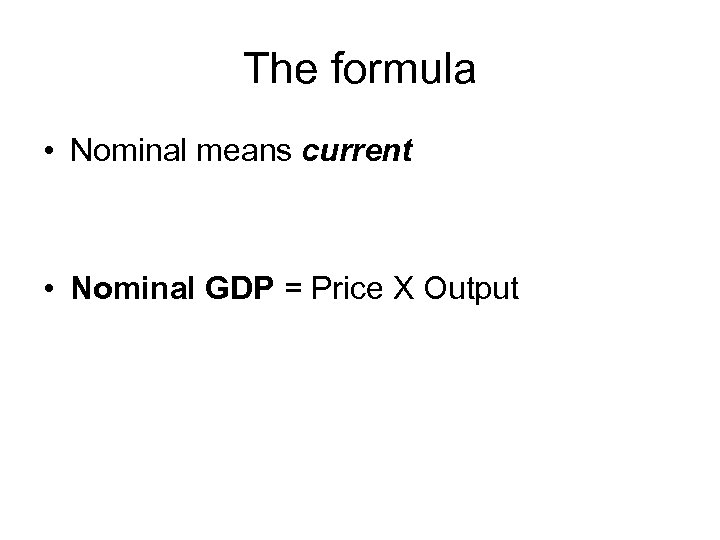The formula • Nominal means current • Nominal GDP = Price X Output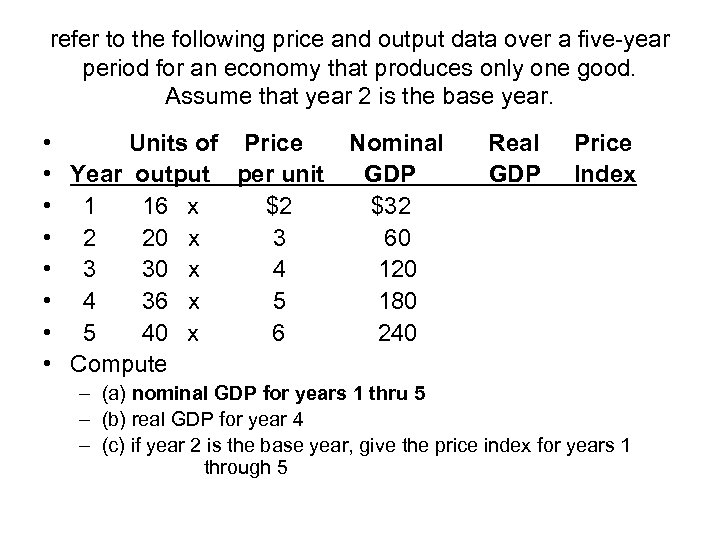refer to the following price and output data over a five-year period for an economy that produces only one good. Assume that year 2 is the base year. • Units of Price • Year output per unit • 1 16 x \$2 • 2 20 x 3 • 3 30 x 4 • 4 36 x 5 • 5 40 x 6 • Compute Nominal GDP \$32 60 120 180 240 Real GDP Price Index – (a) nominal GDP for years 1 thru 5 – (b) real GDP for year 4 – (c) if year 2 is the base year, give the price index for years 1 through 5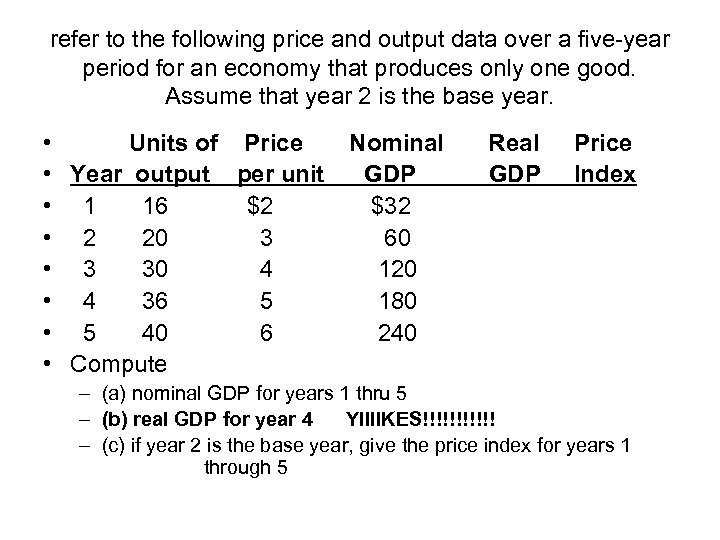refer to the following price and output data over a five-year period for an economy that produces only one good. Assume that year 2 is the base year. • Units of Price • Year output per unit • 1 16 \$2 • 2 20 3 • 3 30 4 • 4 36 5 • 5 40 6 • Compute Nominal GDP \$32 60 120 180 240 Real GDP Price Index – (a) nominal GDP for years 1 thru 5 – (b) real GDP for year 4 YIIIIKES!!!!!! – (c) if year 2 is the base year, give the price index for years 1 through 5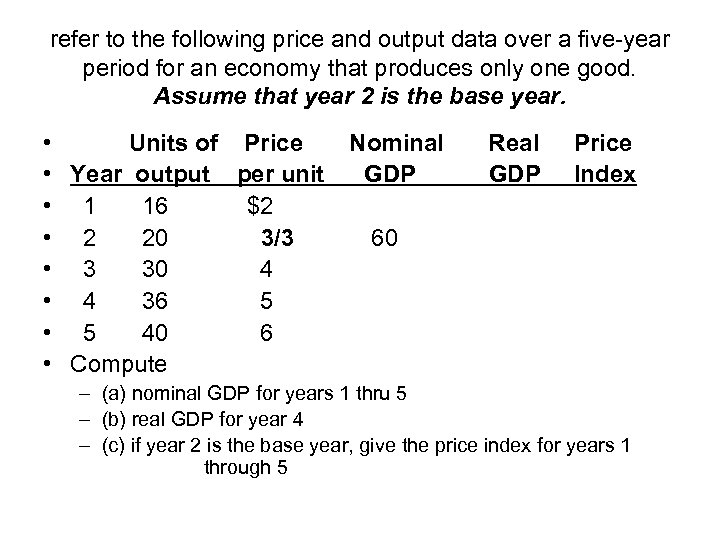refer to the following price and output data over a five-year period for an economy that produces only one good. Assume that year 2 is the base year. • Units of Price • Year output per unit • 1 16 \$2 • 2 20 3/3 • 3 30 4 • 4 36 5 • 5 40 6 • Compute Nominal GDP Real GDP Price Index 60 – (a) nominal GDP for years 1 thru 5 – (b) real GDP for year 4 – (c) if year 2 is the base year, give the price index for years 1 through 5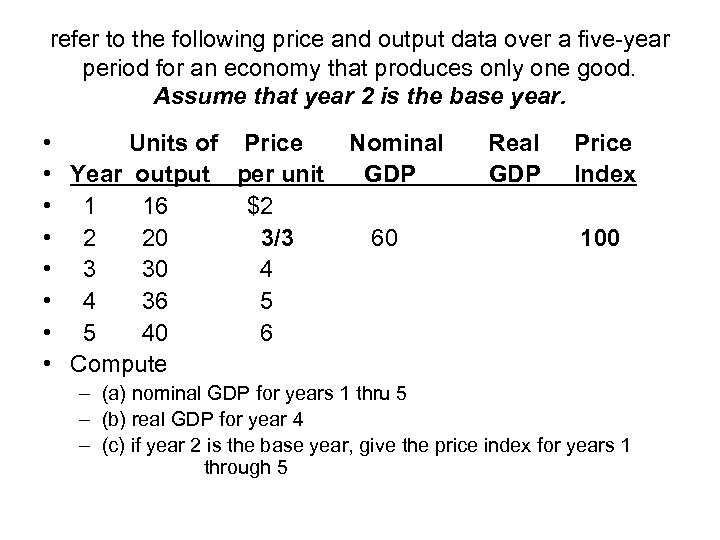refer to the following price and output data over a five-year period for an economy that produces only one good. Assume that year 2 is the base year. • Units of Price • Year output per unit • 1 16 \$2 • 2 20 3/3 • 3 30 4 • 4 36 5 • 5 40 6 • Compute Nominal GDP 60 Real GDP Price Index 100 – (a) nominal GDP for years 1 thru 5 – (b) real GDP for year 4 – (c) if year 2 is the base year, give the price index for years 1 through 5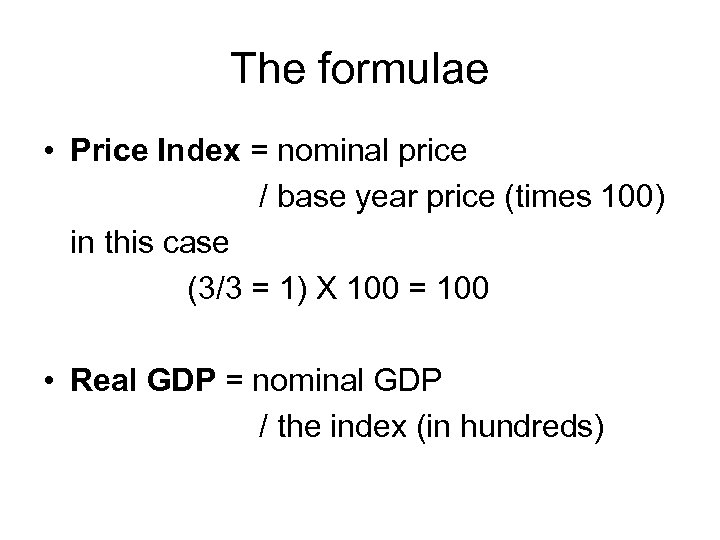The formulae • Price Index = nominal price / base year price (times 100) in this case (3/3 = 1) X 100 = 100 • Real GDP = nominal GDP / the index (in hundreds)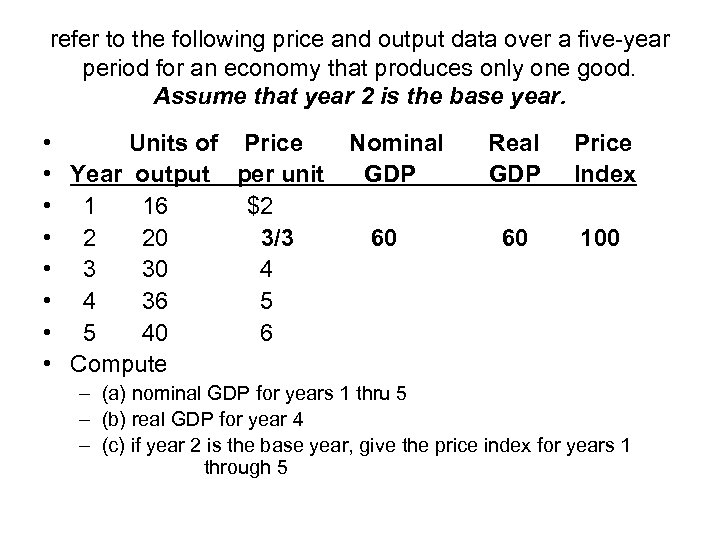refer to the following price and output data over a five-year period for an economy that produces only one good. Assume that year 2 is the base year. • Units of Price • Year output per unit • 1 16 \$2 • 2 20 3/3 • 3 30 4 • 4 36 5 • 5 40 6 • Compute Nominal GDP 60 Real GDP Price Index 60 100 – (a) nominal GDP for years 1 thru 5 – (b) real GDP for year 4 – (c) if year 2 is the base year, give the price index for years 1 through 5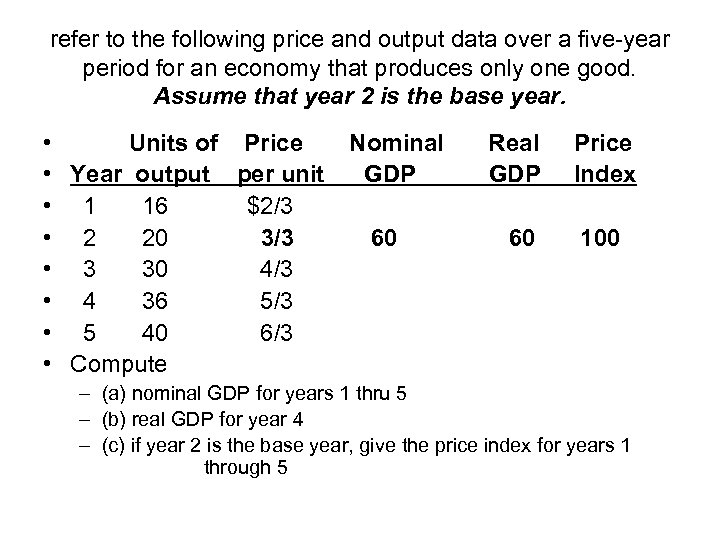refer to the following price and output data over a five-year period for an economy that produces only one good. Assume that year 2 is the base year. • Units of Price • Year output per unit • 1 16 \$2/3 • 2 20 3/3 • 3 30 4/3 • 4 36 5/3 • 5 40 6/3 • Compute Nominal GDP 60 Real GDP 60 Price Index 100 – (a) nominal GDP for years 1 thru 5 – (b) real GDP for year 4 – (c) if year 2 is the base year, give the price index for years 1 through 5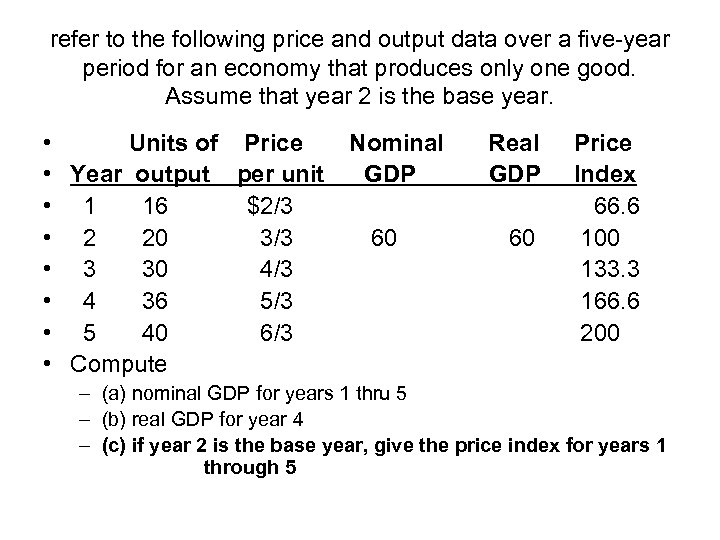refer to the following price and output data over a five-year period for an economy that produces only one good. Assume that year 2 is the base year. • Units of Price • Year output per unit • 1 16 \$2/3 • 2 20 3/3 • 3 30 4/3 • 4 36 5/3 • 5 40 6/3 • Compute Nominal GDP 60 Real GDP 60 Price Index 66. 6 100 133. 3 166. 6 200 – (a) nominal GDP for years 1 thru 5 – (b) real GDP for year 4 – (c) if year 2 is the base year, give the price index for years 1 through 5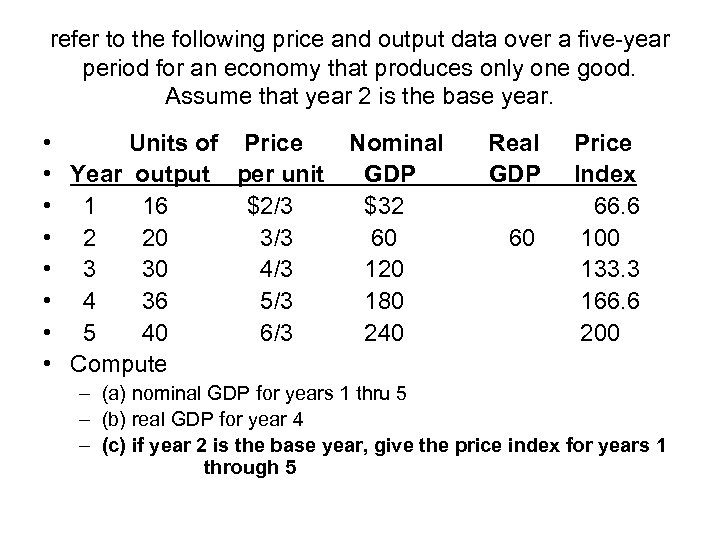refer to the following price and output data over a five-year period for an economy that produces only one good. Assume that year 2 is the base year. • Units of Price • Year output per unit • 1 16 \$2/3 • 2 20 3/3 • 3 30 4/3 • 4 36 5/3 • 5 40 6/3 • Compute Nominal GDP \$32 60 120 180 240 Real GDP 60 Price Index 66. 6 100 133. 3 166. 6 200 – (a) nominal GDP for years 1 thru 5 – (b) real GDP for year 4 – (c) if year 2 is the base year, give the price index for years 1 through 5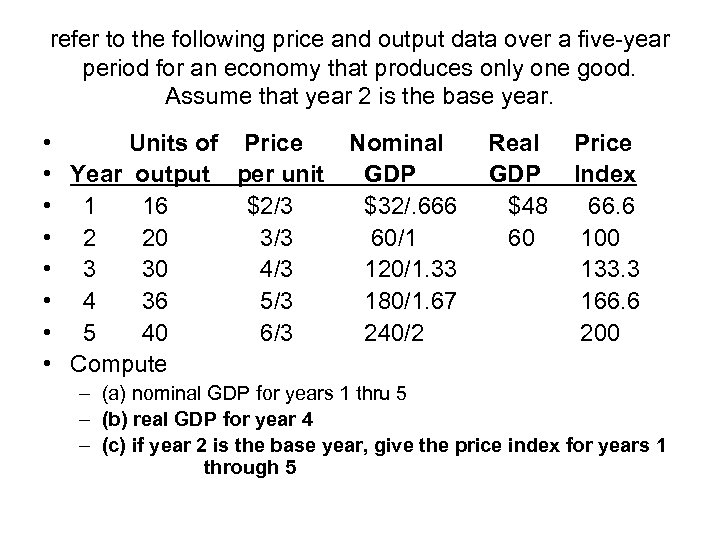refer to the following price and output data over a five-year period for an economy that produces only one good. Assume that year 2 is the base year. • Units of Price • Year output per unit • 1 16 \$2/3 • 2 20 3/3 • 3 30 4/3 • 4 36 5/3 • 5 40 6/3 • Compute Nominal GDP \$32/. 666 60/1 120/1. 33 180/1. 67 240/2 Real GDP \$48 60 Price Index 66. 6 100 133. 3 166. 6 200 – (a) nominal GDP for years 1 thru 5 – (b) real GDP for year 4 – (c) if year 2 is the base year, give the price index for years 1 through 5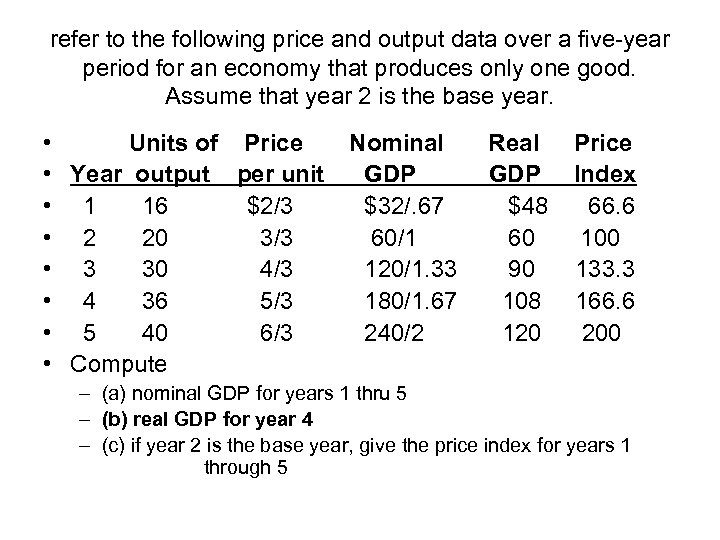refer to the following price and output data over a five-year period for an economy that produces only one good. Assume that year 2 is the base year. • Units of Price • Year output per unit • 1 16 \$2/3 • 2 20 3/3 • 3 30 4/3 • 4 36 5/3 • 5 40 6/3 • Compute Nominal GDP \$32/. 67 60/1 120/1. 33 180/1. 67 240/2 Real GDP \$48 60 90 108 120 Price Index 66. 6 100 133. 3 166. 6 200 – (a) nominal GDP for years 1 thru 5 – (b) real GDP for year 4 – (c) if year 2 is the base year, give the price index for years 1 through 5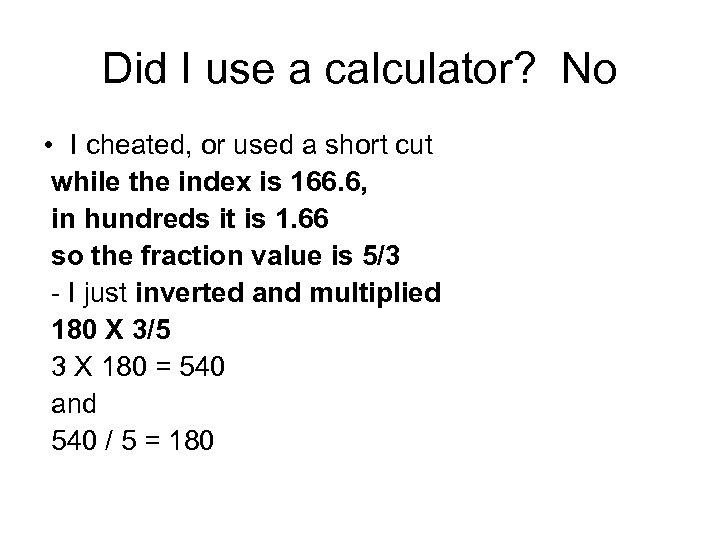Did I use a calculator? No • I cheated, or used a short cut while the index is 166. 6, in hundreds it is 1. 66 so the fraction value is 5/3 - I just inverted and multiplied 180 X 3/5 3 X 180 = 540 and 540 / 5 = 180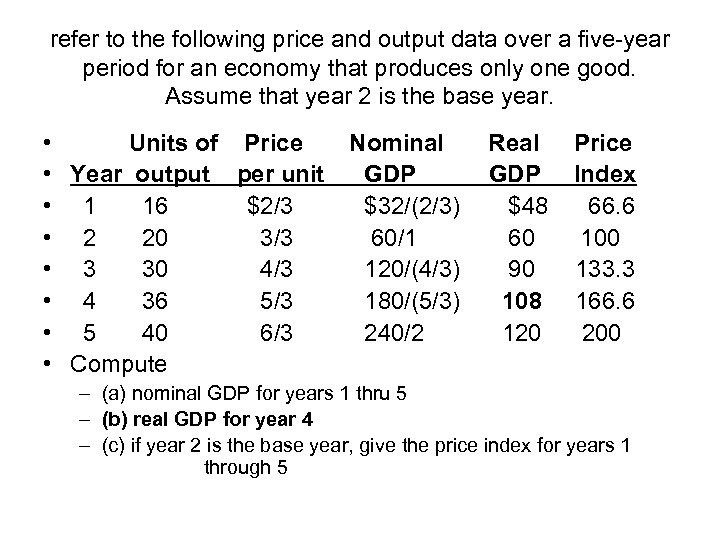refer to the following price and output data over a five-year period for an economy that produces only one good. Assume that year 2 is the base year. • Units of Price • Year output per unit • 1 16 \$2/3 • 2 20 3/3 • 3 30 4/3 • 4 36 5/3 • 5 40 6/3 • Compute Nominal GDP \$32/(2/3) 60/1 120/(4/3) 180/(5/3) 240/2 Real GDP \$48 60 90 108 120 Price Index 66. 6 100 133. 3 166. 6 200 – (a) nominal GDP for years 1 thru 5 – (b) real GDP for year 4 – (c) if year 2 is the base year, give the price index for years 1 through 5